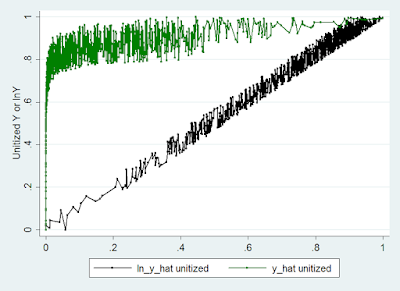## Monday, May 7, 2012

### Estimating Supply* Stata Simulation
* Simulate Commodity Supply
* Classical Microeconomics

* Let's think of the typical firm in classical microeconomics

* Max{x} {Profit funciton =p*f(x)-w*x)}

* In order for there to be an interior solution: f'(x)>0, f''(x)<0

* There is an infinite number of functions that have this property.

* Let's choose a typical one.

* 0
* f(x) = x^(alpha)
* so f'(x) = alpha*x^(alpha-1)
* and Profit funciton' =p*alpha*x^(alpha-1) -w=0
* x^(alpha-1)=w/(p*alpha)
* x=(w/(p*alpha))^(1/(alpha-1))

* Let's imagine a 1000 cities

* Each has a different market price and different input price

clear
set obs 1000
set seed 101

* Declare the parameter of interest
global alpha=.8

gen p = (runiform()+2.3)+1
* hist p
gen w = rnormal()^2+1
* hist w

* Here are two different right skewed distributions of prices
* Firm produced based on:
gen x=(w/(p*\$alpha))^(1/(\$alpha-1))

sum x

* Generate the output as a result of the inputs
* Plus some stochastic production shocks
gen y=x^\$alpha*(runiform()+.5)
* A more challenging fit would be:
* gen y=x^\$alpha*(runiform()^3*4)

sum y, detail

* Most firms produce between 2.5 and 52 units

* Firms make profit:
gen profit = p*y - w*x

sum profit

* First thing, can we recover the marginal product of x
* ln(y) = alpha*ln(x)+ln(runiform()+.5)
* E(ln(y)) = alpha*E(ln(x)) + E(ln(runiform()+.5))
* E(ln(runiform()+.5))<0 since E(runiform()+.5)=1 and ln(1)=0
* by Jensen's inequality E(ln(runiform()+.5))<0
* However, that should not throw off our estimates of alpha

gen lny=ln(y)
gen lnx=ln(x)

reg lny lnx
di _b[lnx] "is pretty close to \$alpha"

* We could also try to estimate this by non-linear least squares
nl (y=x^{alpha})

* This seems to do a pretty good job as well.

* We might however be interested in seeing how supply responds to changes
* in prices.

* let's try a simple OLS
reg y p w

predict y_hat
label var y_hat
line y_hat y, sort
* does not quite look right

* This seems to be a pretty good fit.  Let's try a different approach
gen lnp = ln(p)
gen lnw = ln(w)

reg lny lnp lnw
predict lny_hat

* I want to graph both lny_hat and y_hat on the same graph
* But I will need to unitize everything (distributions to
* start at 0 and end at 1

foreach v in lny_hat lny y_hat y {
sum `v'
gen unit_`v'=(`v'-r(min))/(r(max)-r(min))
}

label var unit_lny_hat "ln_y_hat unitized"
label var unit_y_hat "y_hat unitized"

two (connected unit_lny_hat unit_lny, sort msize(vsmall) color(black)) ///
(connected unit_y_hat unit_y, sort  msize(vsmall) color(green))  , ///
ytitle("Unitized Y or lnY")

* Elasticities however, work even better.  Why is that?
* look first how x is generated
* x=(w/(p*\$alpha))^(1/(\$alpha-1))
* now y=x^\$alpha*(runiform()+.5)
* y=(w/(p*\$alpha))^(1/(\$alpha-1))^\$alpha*(runiform()+.5)
* y=(w/(p*\$alpha))^(1/(\$alpha-1))*\$alpha*(runiform()+.5)
* lny=(1/(\$alpha-1))*\$alpha*(runiform()+.5)ln(w/(p*\$alpha))
* lny=(1/(\$alpha-1))*\$alpha*(runiform()+.5){ln(w) - ln(p) - ln(\$alpha)}
* plim(lny)=(1/(\$alpha-1))*\$alpha*plim((runiform()+.5))plim({ln(w) - ln(p) - ln(\$alpha)})
* plim(lny)=(1/(\$alpha-1))*\$alpha*plim({ln(w) - ln(p) - ln(\$alpha)})
* plim(lny)=(1/(\$alpha-1))*\$alpha*[E{ln(w) - E(ln(p)) - ln(\$alpha)]

di "Therefore (1/(\$alpha-1))*\$alpha = " (1/(\$alpha-1))*\$alpha " ~ " -_b[lnp] " ~ " _b[lnw]
di "And (1/(\$alpha-1))*\$alpha)*-ln(\$alpha) = " (1/(\$alpha-1)*\$alpha)*-ln(\$alpha) " ~ "  _b[_cons] " ?"